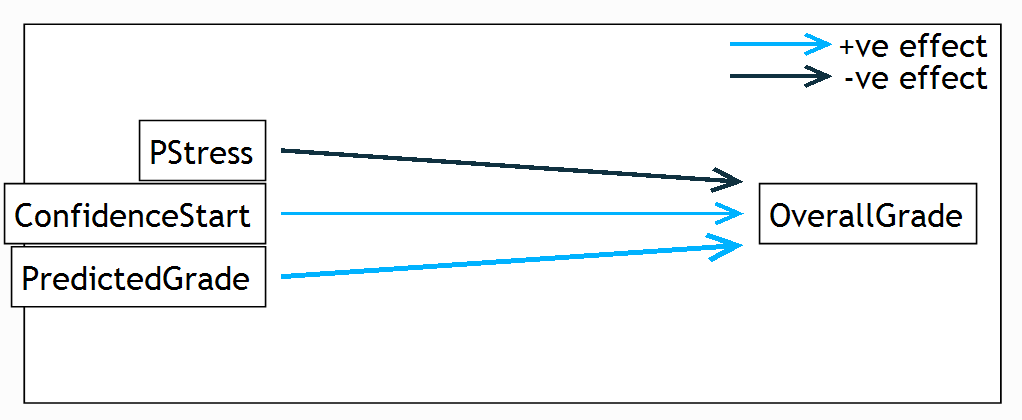1. A continuous (Interval) DV requires the use of a General Linear model.
2. A Categorical DV requires the use of a Generalized Linear model.
3. If you are interested in whether or not a variable is having a unique effect on a DV, then use ANOVA.

Fill in the gaps in the table and in the model formula to complete the results

Below are the results of a GLM analysis looking at three variables that might influence a student’s overall grade. There were 51 participants.OverallGrade = 52 – 0.521 × PStress + 0.01 ×  ConfidenceStart + 0.401 × PredictedGrade +

 Coefficient Estimated value SE t df p-value Intercept 52.5 13.41 n/a 47 n/a PStress –0.521 0.380 –1.37 47 0.174 ConfidenceStart 0.010 0.067 0.15 47 0.882 PredictedGrade 0.401 0.147 2.72 47 0.008